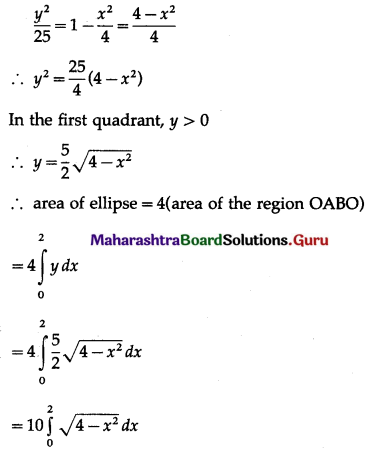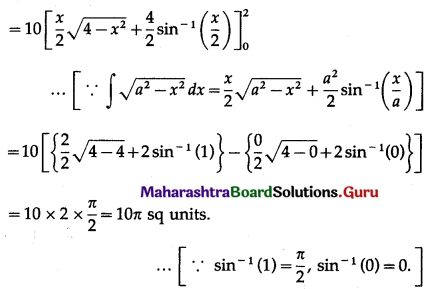Balbharati Maharashtra State Board Std 12 Commerce Statistics Part 1 Digest Pdf Chapter 7 Application of Definite Integration Ex 7.1 Questions and Answers.

## Maharashtra State Board 12th Commerce Maths Solutions Chapter 7 Application of Definite Integration Ex 7.1

Question 1.
Find the area of the region bounded by the following curves, the X-axis, and the given lines:
(i) y = x4, x = 1, x = 5
Solution: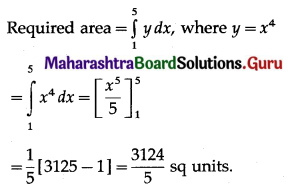(ii) y = $$\sqrt{6 x+4}$$, x = 0, x = 2
Solution: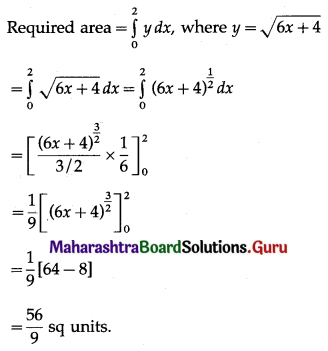(iii) $$\sqrt{16-x^{2}}$$, x = 0, x = 4
Solution: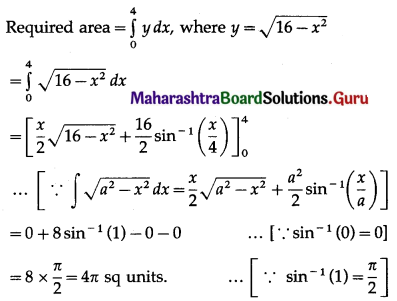(iv) 2y = 5x + 7, x = 2, x = 8
Solution: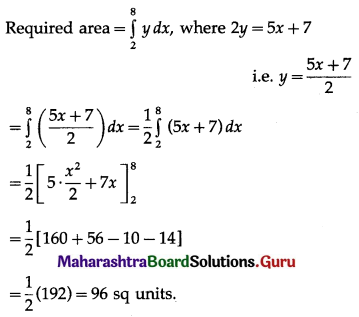(v) 2y + x = 8, x = 2, x = 4
Solution: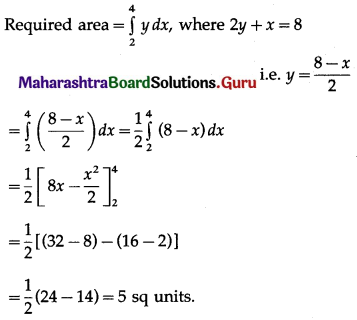(vi) y = x2 + 1, x = 0, x = 3
Solution: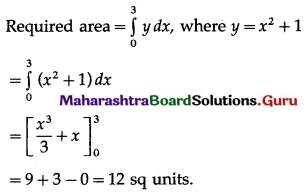(vii) y = 2 – x2, x = -1, x = 1
Solution: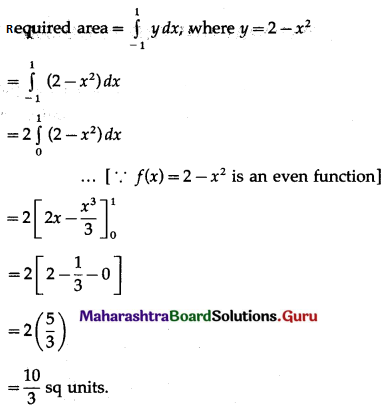Question 2.
Find the area of the region bounded by the parabola y2 = 4x and the line x = 3.
Solution: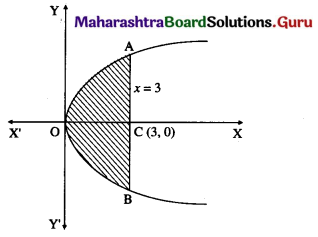Required area = area of the region OABO
= 2(area of the region OACO)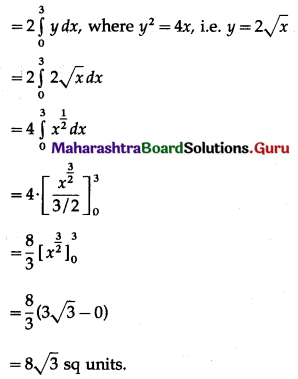Question 3.
Find the area of the circle x2 + y2 = 25.
Solution:By the symmetry of the circle, its area is equal to 4 times the area of the region OABO.
Clearly, for this region, the limits of integration are 0 and 5.
From the equation of the circle, y2 = 25 – x2.
In the first quadrant y > 0
∴ y = $$\sqrt{25-x^{2}}$$
∴ area of the circle = 4(area of region OABO)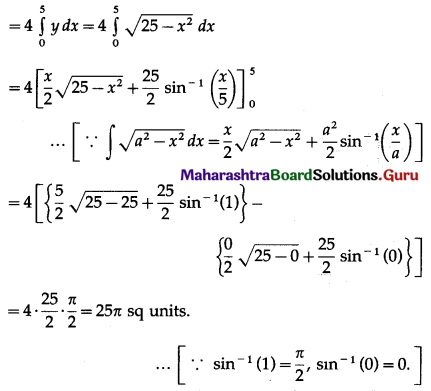Question 4.
Find the area of the ellipse $$\frac{x^{2}}{4}+\frac{y^{2}}{25}$$ = 1
Solution: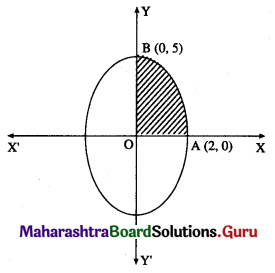By the symmetry of the ellipse, its area is equal to 4 times the area of the region OABO.
Clearly, for this region, the limits of integration are 0 and 2.
From the equation of the ellipse,